# box() Function in R

• Last Updated : 02 Jun, 2022

Box( ) function in R programming is used to draw a box around the current plot in the given color and line type. The function box( ) is built under ‘graphics’ package of R programming. The bty argument of the plotting function determines the type of box drawn.

Syntax: box(which = “plot”, lty = “solid”, …)

Parameters:

• which: character, one of “plot”, “figure”, “inner” and “outer”.
• lty: line type of the box.

Example 1:

We will plot a curve without axes i.e. ‘axes = FALSE’ in order to avoid default boxes and then add a red box to the plot. We will plot a curve of tan(x).

## R

 `# Plotting curve``# without axes``curve``(tan, -20, 20, axes = ``FALSE``)`` ` `# Using box() to draw``# a Red box around``# the curve``box``(which = ``"plot"``,col = 6, lty = ``"solid"``)`

Output :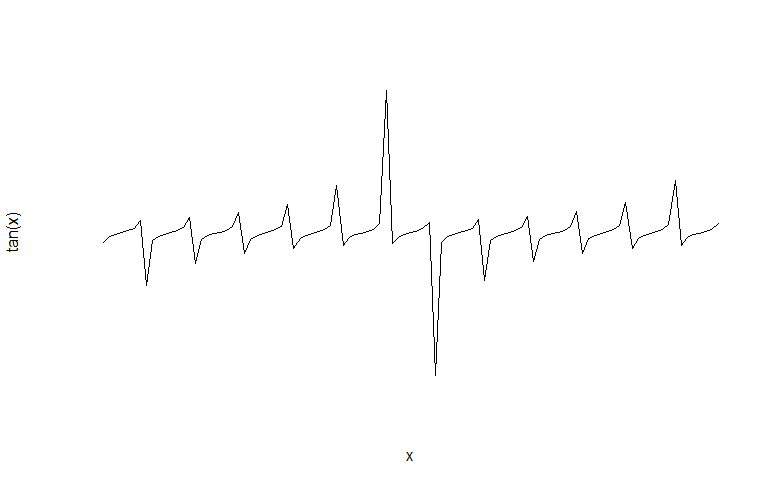Plot without axes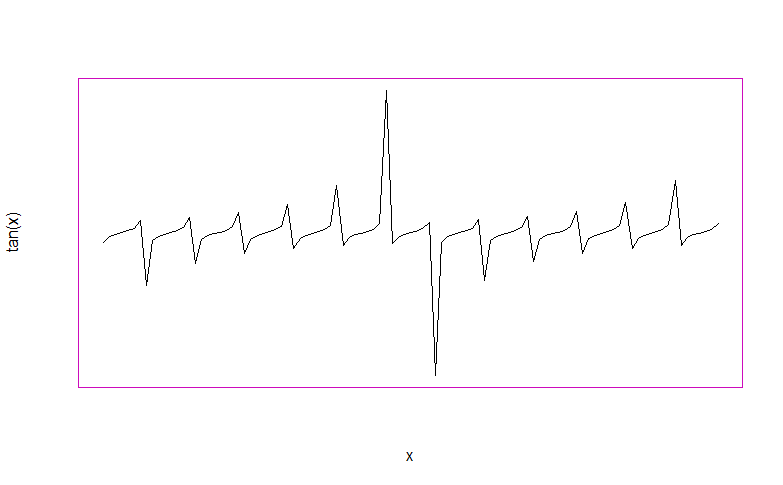Plot enclosed in a box.

### Types of boxes :

The default box around the base R plots can be customized using ‘bty’ argument of the corresponding function. Boxes specified under the argument can be of types :

• Full box – bty = “o” (Default)
• Left and bottom – bty = “L”
• Top, left and bottom – bty = “C”
• Top and right – bty = “7”
• Top, right and bottom – bty = “]”
• Left, bottom and right – bty = “U”
• No box – bty = “n”

Example 2:

We will start with plotting a statistical plot of type line and dot and then well will try to add different types of boxes using ‘bty’ argument.

## R

 `# plotting a line curve``# using plot( ) function.``plot``(1:10, ``abs``(stats::``rnorm``(10)),``     ``type = ``"b"``, axes = ``FALSE``)`` ` `# adding axes and labels``# to the plot``axis``(1, at = 1:10, labels = ``letters``[1:10])`

Output :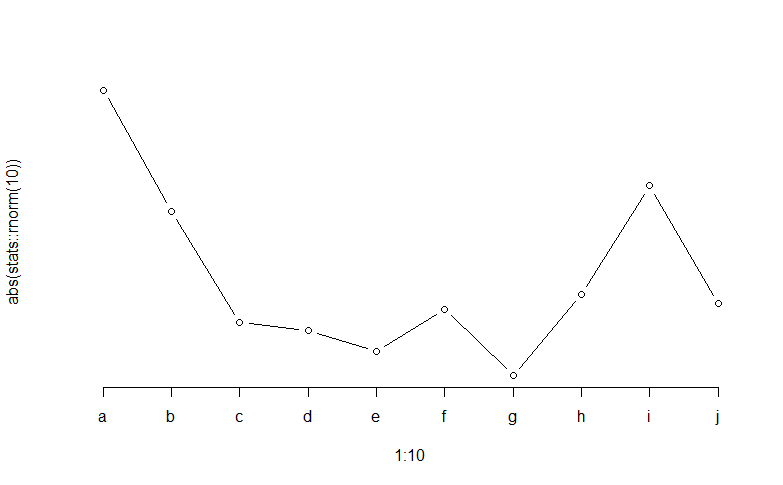Now we will add a box having only left and bottom rules using bty=”L” argument.

## R

 `box``(which=``"plot"``,lty = ``'solid'``, col = ``'blue'``,bty=``"L"``)`

Output :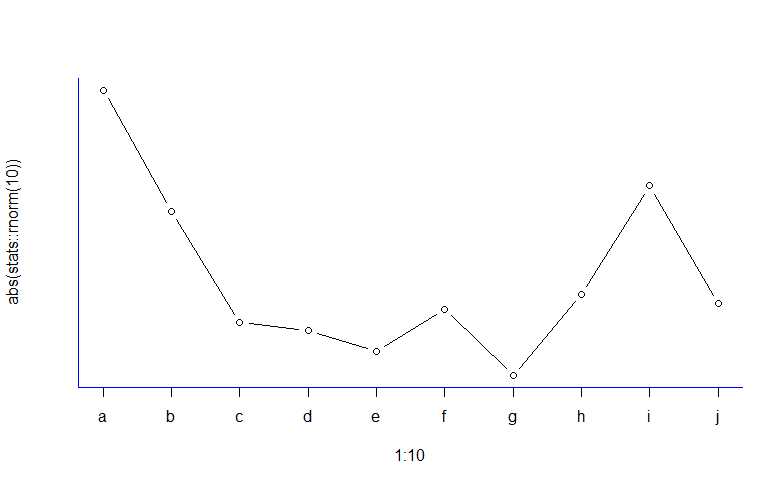Now we will add a box having a top, right, and bottom rules using bty=”]” argument.

## R

 `box``(which=``"plot"``,lty = ``'solid'``, col = ``'blue'``,bty=``"]"``)`

Output :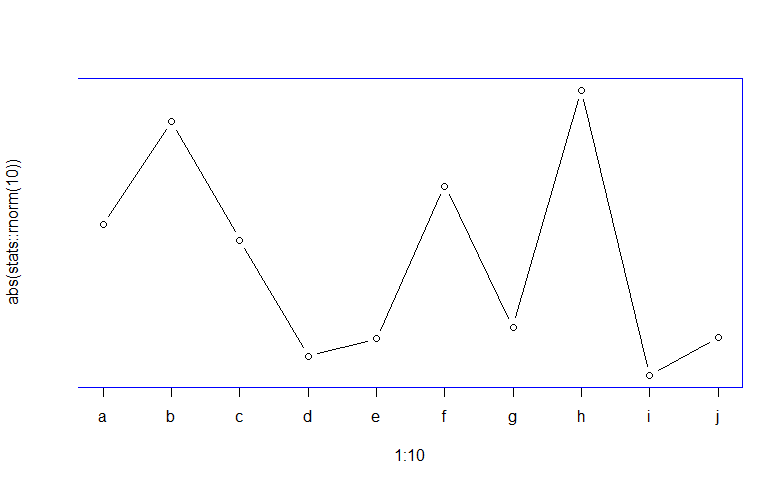My Personal Notes arrow_drop_up